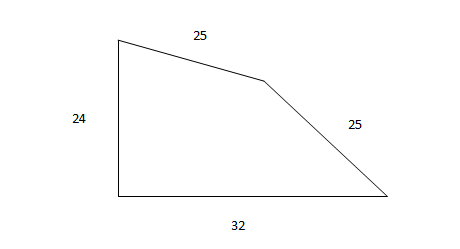Question 27

# Two sides of a plot measure 32 m and 24 m and the angle between them is a perfect right angle. The other two sides measure 25 m each and the other three angles are not right angles. What is the area of the plot?Solution

Length of the diagonal of the right triangle is 40.

The height of the isosceles triangle formed, with 40 as its base is 15.
So, area = (1/2 * 32 * 24) + (1/2 * 40 * 15) = 384 + 300 = 684 $$m^2$$